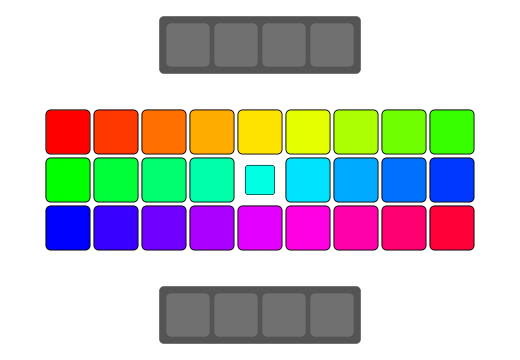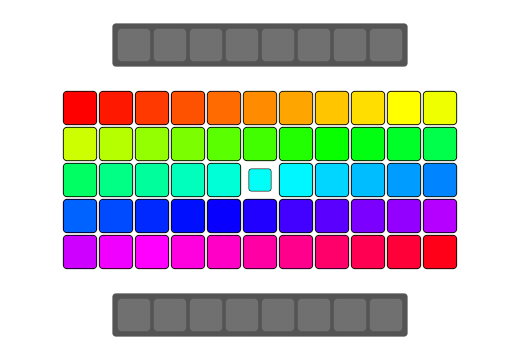C++ compilers these days have code optimization techniques like loop vectorizer which allows the compilers to generate vector instructions for code written in scalar format. It also depends on how the code is written for the compiler to understand if it can auto-vectorize the code or not. In this article I’ll be sharing some ways for developers to guide the compiler to auto-vectorize a certain for loop in C++. It may not necessarily work for all compilers. The examples here are borrowed from ARM’s documentation and applies to following compilers that can generate Neon code:

• GCC, the open source GNU tool-chain.
• LLVM-clang, the open source LLVM-based tool-chain. (Used in Android NDK).
• Arm C/C++ Compiler, designed for Linux user space application development, originally for High Performance Computing.

I gave it a try on Android for a certain code that would convert an 8MP YUV image to ARGB image and observed performance improvements.

## Update - fyi (04/2022)

An easier to read and updated revision of this article has now been published on Medium - Guide the compiler to speed-up your code.

## SIMD, Vectorization and loop unrolling

### SIMD vs SISD

To explain this we first need to talk about modern CPUs

SISD stands for Single Instruction Stream, Single Data Stream. Typically, a program’s code is executed in sequence, i.e. one after another. Let’s say we have two arrays say `a` and `b`, and we want to write a program which converts each element in `a` with following operation:

``````a[i] = a[i] + b[i];
``````

For each index `i` in the arrays. On the other hand, modern CPUs have capabilities and support for SIMD which stands for Single Instruction, Multiple Data. Such machines can exhibit data level parallelism (which is different from concurrency). They can perform the same instruction on multiple data at once. For the above example the SIMD CPUs could group and execute the operations in one batch as:

``````a = a + b;
a = a + b;
a = a + b;
a = a + b;
``````

SIMD instruction for + is called `addps` in SSE or `vaddps` in AVX, and they support grouping of 4 elements and 8 elements respectively (integer type).Figure: SISD swapping - source: dev.toFigure: SIMD swapping - source: dev.to

### Vectorization

SIMD supports instructions that can operate on vector data types. In the above example a group of array elements like `a[0...3]` or `b[4...7]` can be called vectors. Vectorization is the use of vector instructions to speed up program execution. Vectorization can be done both by programmers by explicitly writing vector instructions and by a compiler. The latter case is called `Auto Vectorization`.

Auto Vectorization can be done by AOT (Ahead of Time) compilers at compile time or by JIT (Just in Time) compiler at execution time.

### Loop unrolling

Loop unrolling or Loop unwrapping is a loop transformation technique that attempts to optimize the program’s execution speed at expense of its binary size. The goal of the loop unrolling is to increase a program’s speed by reducing or eliminating instructions that control the loop such as pointer arithmetic and end of loop test on each iteration.

A simple example of loop unrolling would be:

``````// Normal for loop with 16 iterations
for (int i = 0; i < 16000000; ++i) {
a[i] = b[i] + c[i];
}

// Unrolled loop
for (int i = 0; i < 4000000; i+=4) {
a[i] = b[i] + c[i];
a[i+1] = b[i+1] + c[i+1];
a[i+2] = b[i+2] + c[i+2];
a[i+3] = b[i+3] + c[i+3];
}
``````

In the later part of the article, you can see some performance numbers around this.

### Neon

Neon is the implementation of Arm’s Advanced SIMD architecture.

The purpose of Neon is to accelerate data manipulation by providing:

1. Thirty-two 128-bit vector registers, each capable of containing multiple lanes of data.
2. SIMD instructions to operate simultaneously on those multiple lanes of data.

Per documentation there are multiple ways to make use of the technology:

1. Using Neon enabled open source libraries. We all love this!
2. Auto-vectorization feature in the compilers that can take advantage of Neon.
3. Using Neon intrinsics — the compiler will replace them with appropriate Neon instructions. This gives us direct low-level access to the exact Neon instruction we want from `C/C++` code.
4. Writing assembly code with Neon directly, this only works for really well experienced programmers.

In this article we’d be focussing on point#2 and see how we can guide the compiler to auto-vectorize better.

## Guiding compiler to auto-vectorize better

Let’s start with the simple native code in question here, it looks like this:

``````// Code converting YUV image to ARGB. The actual algorithm here is not very important
for (int y = 0; y < image_height; ++y) {
for (int x = 0; x < image_width; ++x) {
int y_idx = (y * y_row_stride) + (x * y_pixel_stride);
y_val = static_cast<int16_t>(y_buffer[y_idx]);

int uvx = x / 2;
int uvy = y / 2;
int uv_idx = (uvy * uv_row_stride) +  (uvx * uv_pixel_stride);

u_val = static_cast<int16_t>(u_buffer[uv_idx]) - 128;
v_val = static_cast<int16_t>(v_buffer[uv_idx]) - 128;

// Compute RGB values per formula above.
r = y_val + 1.370705f * v_val;
g = y_val - (0.698001f * v_val) - (0.337633f * u_val);
b = y_val + 1.732446f * u_val;

int argb_idx = y * image_width + x;
argb_output[argb_idx] = a | r << 16 | g << 8 | b;
}
}
``````

On a Pixel 4a device, converting a `8MP = 3264x2448` YUV image to RGB has the following performance:

# Average latency
Native standard 76.4 ms

Important note: this number was generated on the above code with `-O3` compilation flag. Auto-vectorization is enabled at the -O2, -O3, and -Ofast optimization levels.

### Pragma declaration — `loop vectorize`

Using following `#pragma` declaration just before the for loop indicates to the compiler that the following loop contains no data dependencies that should prevent auto-vectorization:

``````#pragma clang loop vectorize(assume_safety)
``````

Important note: Ensure that you only use this pragma when it is safe to do so it could otherwise lead to race conditions.

So if we fit it into current example:

``````#pragma clang loop vectorize(assume_safety)
for (int y = 0; y < image_height; ++y) {
for (int x = 0; x < image_width; ++x) {
// .. rest of the code as above.
}
}
``````
# Average latency
Native standard 76.4 ms
With `loop vectorize` 68.6 ms

### Pragma declaration — `loop unroll`

Similarly, we can instruct the compiler to unroll loops when compiling with following statement:

``````#pragma clang loop unroll_count(2)
``````

So if we fit it into current example:

``````#pragma clang loop vectorize(assume_safety)
for (int y = 0; y < image_height; ++y) {
#pragma clang loop unroll_count(4)
for (int x = 0; x < image_width; ++x) {
// .. rest of the code as above.
}
}
``````

We get following latency:

# Average latency
Native standard 76.4 ms
With `loop vectorize` 68.6 ms
With `loop vectorize` + `loop unroll_count(4)` 64.5 ms

### Some other tips:

1. Prefer using `<` to construct loops as compared to `<=` or `!=`.
2. Using the `-ffast-math` option can significantly improve the performance of generated code, as far as the algorithm is tolerant to potential inaccuracies as it breaks compliance with IEEE and ISO standards for math operations.

## Closing point

These may not always result in faster code as it depends on how independent the data is w.r.t algorithms. Best case would be to give these a try and benchmark. The performance boost like 18% speedup in this case is definitely worth it.